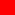## KALYAN CITY LIFE

Sharing Wisdom and Vivid Memories of Life

# Purchasing Power Parity PPP Theory - Gustav Cassel

##Introduction - Purchasing Power Parity PPP Theory

The first original reference of PPP Theory was made by David Ricardo. However, Gustav Cassel popularized this theory in 1918. According to PPP theory, when exchange rates are of a fluctuating nature, the rate of exchange between two currencies in the long run will be fixed by their respective purchasing powers in their own nations.

Gustav Cassel has presented the PPP theory in two versions.

##Absolute Version of the PPP Theory

According to the absolute version of the purchasing power parity (PPP) theory, the exchange rates between two currencies should reflect the relation between the international purchasng powers of various currencies. In simple words the exchange rate would be determined, at the point where the internal purchasing power of the respective currencies gets equalized. Let us take an example to illustrate the point. Suppose particular basket of goods cost Rs. 1000/- in India and \$ 100 in the U.S.A. That means the exchanges rate would be Rs. 10 = \$1.

The exchange rate an be determined with the following equation.

In this equation 'P' i.e. prices are related to the respective bundle of goods with same weights assigned in both the countries. Thus, the above equation explains that the equilibrium exchange rate is determined by the ratio of the internal purchasing power of foreign currency and domestic currency in their own countries. Thus, to conclude the absolute version of this theory maintains the the absolute version of this theory maintains that the absolute purchasing power of respective currencies does play a vital role in determining the equilibrium exchange rate.

##Relative Version of the PPP Theory

The relative version was put forward by Cassel in order to find the strength of the changes in the equilibrium exchange rate. Any departure from the equilibrium will lead to the disequilibrium. It can take place due to changes in the internal purchasing power of a particular currency. The changes in the purchasing power are measured with the help of domestic price indices if the respective nation. We need to assume any past rate of exchange as a base exchange rate in order to know the percentage change in the exchange rate. If we compare the price indices in the past i.e. base period with that of the present period, the new equilibrium exchange rate could be found out.

It can be simplified with the following equation.

Thus, according to the equation when the price level in concerned nation changes, automatically the internal purchasing power of the currency of that nation goes on changing. This change leads to the change in the equilibrium exchange rate. Thus, under this theory Gustav Cassel has tried to link the purchasing power of two currencies in determining the equilibrium exchange rate. However, it has been criticized on the following grounds.

##Criticism of Purchasing Power Parity (PPP) Theory

1. Limitations of the Price Index : As seen above in the relative version the PPP theory uses the price index in order to measure the changes in the equilibrium rate of exchange. However, price indices suffer from various limitations and thus theory too.
2. Neglect of the demand / supply approach : The theory fails to explain the demand for as well as the supply of foreign exchange. The PPP theory proves to be unsatisfactory due to this negligence. Because in actual practice the exchange rate is determined according to the market forces such as the demand for and supply of foreign currency.
3. Unrealistic Approach : Since the PPP theory uses price indices which itself proves to be unrealistic. The reason for this is that the quality of goods and services included in the indices differs from nation to nation. Thus, any comparison without due significance for the quality proves to be unrealistic.
4. Unrealistic Assumptions : It is yet another valid criticism that the PPP theory is based on the unrealistic assumptions such as absence of transport cost. Also it wrongly assumes that there is an absence of any barriers to the international trade.
5. Neglects Impact of International Capital Flow : The PPP theory neglects the impact of the international capital movements on the foreign exchange market. International capital flows may cause fluctuations in the existing exchange rate.
6. Rare Occurrence : According to critics, the PPP theory is in contrast to the Practical approach. Because, the rate of exchange between any two currencies based on the domestic price ratios is a very rare occurrence.
Thus, the PPP theory is criticized on the above grounds.

##Conclusion On Purchasing Power Parity Theory

Despite these criticisms the theory focuses on the following major points.

1. It tries to establish relationship between domestic price level and the exchange rates.
2. The theory explains the nature of trade as well as considers the BOP (Balance of Payments) of a nation.

Thus, Gustav cassell's attempt to explain the exchange rate determination based on domestic price indices was very unique attempt.

## Sitemap of Kalyan City Life Blog

### 1 Comment:

1. Anonymous said...

Really nice article with easy language!! Thanks